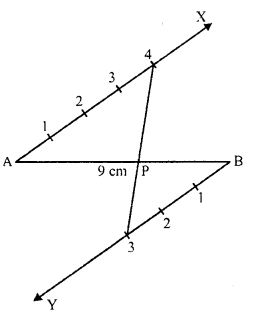## RD Sharma Class 10 Solutions Chapter 9 Constructions Ex 9.1

These Solutions are part of RD Sharma Class 10 Solutions. Here we have given RD Sharma Class 10 Solutions Chapter 9 Constructions Ex 9.1

Other Exercises

Question 1.
Determine a point which divides a line segment of length 12 cm internally in the ratio 2 : 3. Also justify your construction.
Solution:
Steps of construction :
(i)
Draw a line segment AB = 12 cm.
(ii) Draw a ray AX at A making an acute angle with AB.
(iii) From B, draw another ray BY parallel to AX.
(iv) Cut off 2 equal parts from AX and 3 equal parts from BY.
(v) Join 2 and 3 which intersects AB at P.
P is the required point which divides AB in the ratio of 2 : 3 internally.Question 2.
Divide a line segment of length 9 cm internally in the ratio 4 : 3. Also, give justification of the construction.
Solution:
Steps of construction :
(i)
Draw a line segment AB = 9 cm.
(ii) Draw a ray AX making an acute angle with AB.
(iii) From B, draw another ray BY parallel to AX.
(iv) Cut off 4 equal parts from AX and 3 parts from BY.
(v) Join 4 and 3 which intersects AB at P.
P is the required point which divides AB in the ratio of 4 : 3 internally.Question 3.
Divide a line segment of length 14 cm internally in the ratio 2 : 5. Also justify your construction.
Solution:
Steps of construction :
(i)
Draw a line segment AB = 14 cm.
(ii) Draw a ray AX making an acute angle with AB.
(iii) From B, draw another ray BY parallel to AX.
(iv) From AX, cut off 2 equal parts and from B, cut off 5 equal parts.
(v) Join 2 and 5 which intersects AB at P.
P is the required point which divides AB in the ratio of 2 : 5 internally.Question 4.
Draw a line segment of length 8 cm and divide it internally in the ratio 4 : 5.
Solution:
Steps of construction :
(i)
Draw a line segment AB = 8 cm.
(ii) Draw a ray AX making an acute angle with ∠BAX = 60° withAB.(iii) Draw a ray BY parallel to AX by making an acute angle ∠ABY = ∠BAX.
(iv) Mark four points A1, A2, A3, A4 on AX and five points B1, B2, B3, B4, Bs on BY in such a way that AA1 = A1A2 = A2A3 = A3A4 .
(v) Join A4B5.
(vi) Let this line intersect AB at a point P.
Thus, P is the point dividing the line segment AB internally in the ratio of 4 : 5.

Hope given RD Sharma Class 10 Solutions Chapter 9 Constructions Ex 9.1 are helpful to complete your math homework.

If you have any doubts, please comment below. Learn Insta try to provide online math tutoring for you.